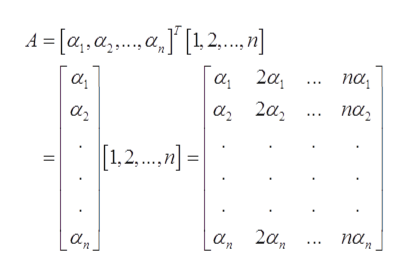# Let vectors a = [ α 1 , α 2 , … , α n ] a n d b = [ 1 , 2 , … , n ] and form a matrix  A = a T b .  Select all of the following statements that are correct.Group of answer choicesa) A is nxn in sizeb) det(A) = 0c) We cannot determine the rank of Ad) A is singulare) We cannot determine the determinant of Af) rank(A) = 1g) A is 1x1 in sizeh) rank(A) = n

Question
26 views

Let vectors a = [ α 1 , α 2 , … , α n ] a n d b = [ 1 , 2 , … , n ] and form a matrix  A = a T b .

Select all of the following statements that are correct.

a) A is nxn in size
b) det(A) = 0
c) We cannot determine the rank of A
d) A is singular
e) We cannot determine the determinant of A
f) rank(A) = 1
g) A is 1x1 in size
h) rank(A) = n
check_circle

Step 1

Since we only answer up to 3 sub-parts, we’ll answer the first 3. Please resubmit the question and specify the other subparts (up to 3) you’d like answered.

Given vectors are,

Step 2

Now, matrix A is,help_outlineImage Transcriptionclose4- [а, а,.а, f [12. а, 2а, а, па, а, 2а, па, 1,2 = а, 2а, па, а, fullscreen
Step 3

Since, dimension of transpose of a is nx1 and dimension of b is 1xn.

Hence, the dimen...

### Want to see the full answer?

See Solution

#### Want to see this answer and more?

Solutions are written by subject experts who are available 24/7. Questions are typically answered within 1 hour.*

See Solution
*Response times may vary by subject and question.
Tagged in